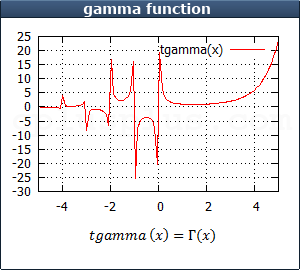function
<cmath> <ctgmath>

# tgamma

```     double tgamma  (     double x);
float tgammaf (      float x);
long double tgammal (long double x);
```
```     double tgamma (     double x);
float tgamma (      float x);
long double tgamma (long double x);
double tgamma (T x);           // additional overloads for integral types```
Compute gamma functionReturns the gamma function of x.

Header <tgmath.h> provides a type-generic macro version of this function.
Additional overloads are provided in this header (`<cmath>`) for the integral types: These overloads effectively cast x to a `double` before calculations (defined for T being any integral type).

### Parameters

x
Parameter for the gamma function.

### Return Value

Gamma function of x.
If the magnitude of x is too large, an overflow range error occurs. If too small, an underflow range error may occur.
If x is zero or a negative integer for which the function is asymptotic, it may cause a domain error or a pole error (or none, depending on implementation).

If a domain error occurs:
- And math_errhandling has MATH_ERRNO set: the global variable errno is set to EDOM.
- And math_errhandling has MATH_ERREXCEPT set: FE_INVALID is raised.

If a range error occurs:
- And math_errhandling has MATH_ERRNO set: the global variable errno is set to ERANGE.
- And math_errhandling has MATH_ERREXCEPT set: either FE_OVERFLOW or FE_UNDERFLOW is raised.

If a pole error occurs:
- And math_errhandling has MATH_ERRNO set: the global variable errno is set to ERANGE.
- And math_errhandling has MATH_ERREXCEPT set: either FE_DIVBYZERO is raised.

### Example

 ``123456789101112`` ``````/* tgamma example */ #include /* printf */ #include /* tgamma */ int main () { double param, result; param = 0.5; result = tgamma (param); printf ("tgamma(%f) = %f\n", param, result ); return 0; }``````

Output:
 ``` tgamma (0.500000) = 1.772454 ```